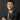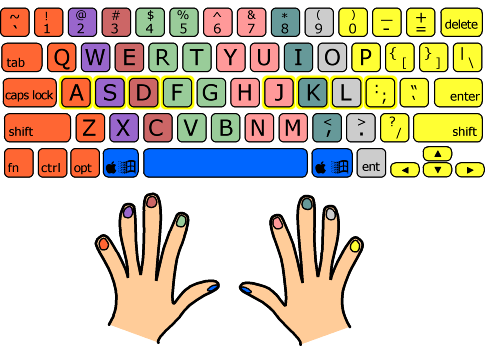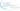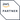# 學好手勢 == 學好CodingGordon Lau 劉偉中
2019-08-21SourceSource

## 編程手勢

```.css-qhxz92{max-width:100%;}```/*第一段*/
function sum(a){
var total = 0;
for(var i = 0 ; i< a.length ; i++){
total = i + total;
}
}``````

``````/*第二段*/
function sum(array){
let total  = 0;
for(let i = 0; i< a.length ; i++){
total = i +total;
}
}``````

``````/*第三段*/
function sum(array){
let total = 0;
for(let num of a){
total += num;
}
}``````

``````/*第四段*/
const sum = array=>array.reduce((acc,num)=>acc+num)``````

``````function sum(array){
/*For-Loop*/
}``````

``const sum = array=>/*Reduce method*/``

## 閱讀更多## 新年願望：學寫程式懶人包Gordon Lau 劉偉中
2019-01-02## 專家級新手Gordon Lau 劉偉中
2019-03-19## 軟件工程師成長手冊Gordon Lau 劉偉中
2019-04-29

##### 索取課程大綱
###### 提交後, 請檢查你的電郵